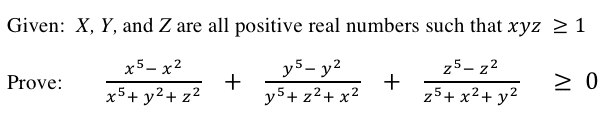# Exponent Proof

physicsguy101

## Homework Statement

I've typed up in Word (it's easier for me to make equations in there) and uploaded as an image below a problem I came across, and I'm curious as to why this works. Obviously, since x,y,z are all positive numbers, if the numbers plugged in are all greater than 1, then it's simple. But x,y, and/or z can also be decimals. I'm curious to know why it works with these decimals as well, when raising to a higher power makes the value smaller.

If anyone can help me write up the proof, I would greatly appreciate it. Thanks in advance.Homework Helper
You mean for 0<x,y,z<1 ... "decimal" is a representation of a number, not an actual number.

In this case, you have noticed that x^n < x where 0<x<1. But it is still a positive number. There is no value of n which will make x^n negative.

You next problem would be that x^m - x^n < 1 if m > n and 0 < x < 1.
I'm guessing this is what you are talking about. You are right - the proof for x > 1 does not work in this range.

That's fine - you don't need it to work over this range. You are explicitly told to prove the case for x,y,z ≥ 1 ... unless you did not misstype and you need only the product of the three numbers is xyz ≥ 1

If so then consider: this product means that all three are positive or just one is positive. Further, the product cannot lie between zero and one.
For instance, I could pick all three x=y=z=0.5 so that the relation is not true ... but the product 0.5x0.5x0.5 is less than 1 so the choice does not count.
If x=y=0.5 then z has to be at least 4 ... plug the numbers in and the relation is true!

What you need to show is that even though one or two terms may be negative, the final term/s must provide an even bigger positive number.

arpeggio
Decimals will work because even though the higher power would mean getting smaller, it would NOT become negative,
It can get very close to zero but it will never become a negative number

hence the equation in bigger or equal to zero would work

physicsguy101
Thanks for the responses!

I do understand the decimal cases now, and it all makes sense.

Thanks again.

Harrisonized
First, because of the symmetric nature of this equation, we can factor every term. I'm going to go ahead and factor out the numerator of the first term.

(x5-x2) = x2(x3-1) = x2(x-1)(x2+x+1)

Then the first term will become this:

x2(x-1)(x2+x+1)/(x5+y2+z2)

If x is positive, then x2(x2+x+1) is also positive. (Also notice that these terms shrink quickly as you approach 0.) The problem reduces to solving whether

f(x,y,z) = (x-1)/(x5+y2+z2) + (y-1)/(x2+y5+z2) + (z-1)/(x2+y2+z5)

is positive. What makes you think that f(x,y,z) is positive in the interval 0≤{x,y,z}≤1?

Take the limit of the above as x, y, and z approach 0. You can make the transformation x=y=z in the limit since they all approach the same value, so

limx.y,z→0 f(x,y,z)
= limx→0 3(x-1)/(x5+x2+x2)
= limx→0 3(x-1)/[x2(x3+2)]
= limx→0 3/[x(x3+2)]-3/[x2(x3+2)]
(A polynomial of degree 5 grows faster than a polynomial of degree 4.)
= -∞

You're trying to prove something that's not true. Of course you're going to get stuck. Also notice that f(1,1,1) = 0, so you know that something is going to happen at (1,1,1). What happens is the following:

If (1,1,1) is a local minimum, then there is the possibility that the function is completely positive for (x,y,z)≥0. If not, then there are some points around (1,1,1) that are greater than zero and some points that are less than zero. I'll leave you to prove this for your general case, since you seem like you're the curious type. After proving this you will know why the conclusion you drew in the first post was erroneous.

Last edited: